﻿ 变化环境下水文模型时变参数的识别及函数型式构建 Identifying Functional Form of Time-Varying Hydrological Model Parameters under Changing Environment

Journal of Water Resources Research
Vol.07 No.04(2018), Article ID:26357,9 pages
10.12677/JWRR.2018.74039

Identifying Functional Form of Time-Varying Hydrological Model Parameters under Changing Environment

Mengsi Xiong1, Pan Liu1*, Chao Deng2

1State Key Laboratory of Water Resources and Hydropower Engineering Science, Wuhan University, Wuhan Hubei

2State Key Laboratory of Hydrology-Water Resources and Hydraulic Engineering, Hohai University, Nanjing Jiangsu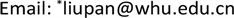Received: Jul. 23rd, 2018; accepted: Aug. 4th, 2018; published: Aug. 13th, 2018ABSTRACT

A two-parameter monthly water balance model was chosen to establish the functional forms of model parameters. The ensemble Kalman filter technique was used first to identify the time-varying parameters, following the construction of the linear functions of parameters and precipitation, potential evapotranspiration and temperature via the correlation analysis between model parameters and climatic factors. The case study was carried out in three Model Parameter Estimation Experiment (MOPEX) catchments in USA using the hydrological data from 1983 to 2003, in which the periods from 1999 to 2001 were selected as droughty year, while 2003 was selected as wet year. The results indicated that: 1) The accuracy of runoff simulations was improved when considering the model parameters as time-varying, especially in peak flows. The Nash-Sutcliffe efficiency coefficient of runoff was increased by 18% and 17% for the calibration and validation periods, respectively; 2) the change of runoff was underestimated when considering the model parameters as constant, with the relative error being up to 38%, while its value was reduced to 7% when considering the model parameters as time-varying. Therefore, taking the hydrological model parameters as a function of climate factors led to a significant enhancement in runoff prediction under changing environment.

Keywords:Changing Environment, Time-Varying Parameters, Parameter Identification, Functional Form

1武汉大学水资源与水电工程科学国家重点实验室，湖北 武汉

2河海大学水文水资源与水利工程国家重点实验室，江苏 南京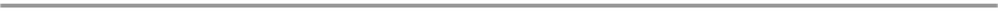1. 引言

2. 研究方法

2.1. 两参数月水量平衡模型

$E{T}_{t}=C×PE{T}_{t}×\mathrm{tanh}\left({P}_{t}/PE{T}_{t}\right)$ (1)

${Q}_{t}={S}_{t}×\mathrm{tanh}\left({S}_{t}/SC\right)$ (2)

${Q}_{t}=\left({S}_{t-1}+{P}_{t}-E{T}_{t}\right)×\mathrm{tanh}\left[\left({S}_{t-1}+{P}_{t}-E{T}_{t}\right)/SC\right]$ (3)

${S}_{t}={S}_{t-1}+{P}_{t}-E{T}_{t}-{Q}_{t}$ (4)

2.2. 集合卡尔曼滤波算法识别时变参数

$\left(\begin{array}{c}{\theta }_{i,k+1}^{f}\\ {x}_{i,k+1}^{f}\end{array}\right)=\left(\begin{array}{c}{\theta }_{i,k}^{a}\\ f\left({x}_{i,k}^{a},{\theta }_{i,k+1}^{f}\right)\end{array}\right)+\left(\begin{array}{c}{\delta }_{i,k}\\ {\epsilon }_{i,k}\end{array}\right),\text{}{\delta }_{i,k}~N\left(0,{R}_{k}\right),{\epsilon }_{i,k}~N\left(0,{G}_{k}\right)$ (5)

${y}_{i,k+1}=h\left({x}_{k+1},{\theta }_{k+1}\right)+{\xi }_{i,k+1},\text{}{\xi }_{i,k+1}~N\left(0,{S}_{k}\right)$ (6)

$\left(\begin{array}{c}{\theta }_{i,k+1}^{a}\\ {x}_{i,k+1}^{a}\end{array}\right)=\left(\begin{array}{c}{\theta }_{i,k+1}^{f}\\ {x}_{i,k+1}^{f}\end{array}\right)+\left(\begin{array}{c}{K}_{k+1}^{\theta }\left[{y}_{i,k+1}-h\left({x}_{i,k+1}^{f},{\theta }_{i,k+1}^{f}\right)\right]\\ {K}_{k+1}^{x}\left[{y}_{i,k+1}-h\left({x}_{i,k+1}^{f},{\theta }_{i,k+1}^{f}\right)\right]\end{array}\right)$ (7)

$\left(\begin{array}{c}{C}_{i,k+1}^{f}\\ S{C}_{i,k+1}^{f}\\ {S}_{i,k+1}^{f}\end{array}\right)=\left(\begin{array}{c}{C}_{i,k}^{a}\\ S{C}_{i,k}^{a}\\ f\left({S}_{i,k}^{a},{C}_{i,k+1}^{f},S{C}_{i,k+1}^{f}\right)\end{array}\right)+\left(\begin{array}{c}{\delta }_{1,i,k}\\ {\delta }_{2,i,k}\\ {\epsilon }_{i,k}\end{array}\right)$ (8)

$\left(\begin{array}{c}{Q}_{i,k+1}\\ E{T}_{i,k+1}\end{array}\right)=\left(\begin{array}{c}h\left({S}_{i,k+1}^{f},{C}_{i,k+1}^{f},S{C}_{i,k+1}^{f}\right)\\ h\left({C}_{i,k+1}^{f}\right)\end{array}\right)+\left(\begin{array}{l}{\xi }_{1,i,k+1}\\ {\xi }_{2,i,k+1}\end{array}\right)$ (9)

2.3. 参数时变型式的确定

$\begin{array}{l}C=f\left(P,T\right)\\ SC=f\left(P,PET,T\right)\end{array}$ (10)

2.4. 评价指标

$RMSE=\sqrt{\frac{1}{n}{\sum }_{t=1}^{n}{\left({Q}_{sim,t}-{Q}_{obs,t}\right)}^{2}}$ (11)

$NSE=1-\frac{\underset{t=1}{\overset{n}{\sum }}{\left({Q}_{sim,t}-{Q}_{obs,t}\right)}^{2}}{\underset{t=1}{\overset{n}{\sum }}{\left({Q}_{obs,t}-{\stackrel{¯}{Q}}_{obs}\right)}^{2}}$ (12)

$RE=\frac{{\stackrel{¯}{Q}}_{sim}-{\stackrel{¯}{Q}}_{obs}}{{\stackrel{¯}{Q}}_{obs}}$ (13)

3. 研究实例

3.1. 流域概况

3.2. 参数时变型式的确定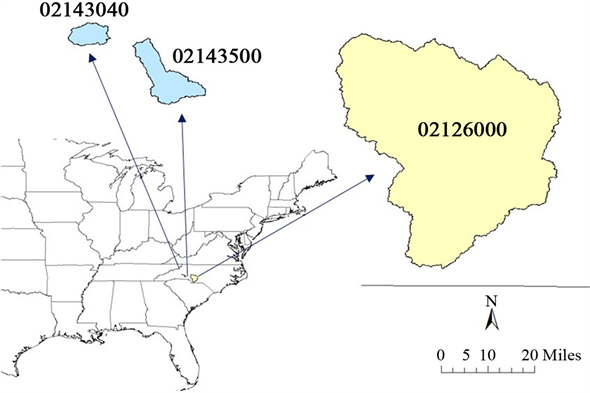Figure 1. Location of the three study basinsTable 1. Basic characteristics of the three basins

$\begin{array}{l}C\left(t\right)={\alpha }_{0}+{\alpha }_{1}T\left(t\right)+{\alpha }_{2}P\left(t-1\right)\\ SC\left(t\right)={\beta }_{0}+{\beta }_{1}PET\left(t\right)+{\beta }_{2}P\left(t\right)+{\beta }_{3}P\left(t-3\right)+{\beta }_{4}T\left(t-3\right)\end{array}$ (14)

3.3. 考虑参数为时变型式的径流模拟

3.4. 时变参数方案下降水变化对径流的影响

6%以内。因此，选取1999~2001三年为干旱年，2003年为湿润年来研究降水变化对径流的影响。表4给出了三个流域在所选干旱年和湿润年的降水径流变化情况，可以看出径流对降水的变化十分敏感，降水变化率60%~80%造成的径流变化率可达300%以上。Table 2. The functional forms of time-varying scheme for two-parameter monthly water balance modelTable 3. Performance statistics for runoff estimates in the calibration and validation periods under different time-varying parameter schemes

4. 结语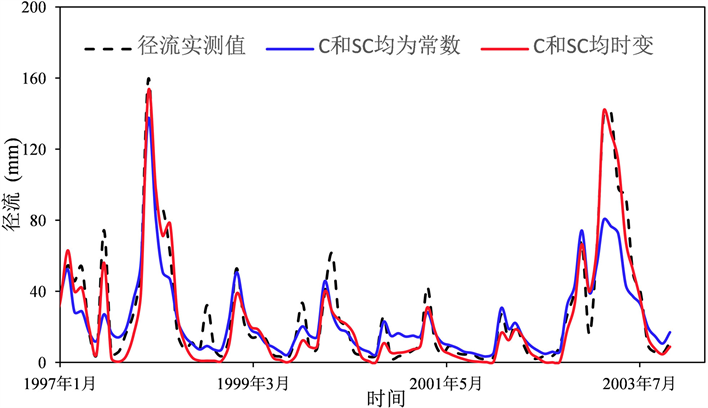Figure 2. The runoff of observed (dashed line) and simulated (solid lines) for time-invariant (blue) and time-varying parameters (red) from 1997-2003 in catchment 02126000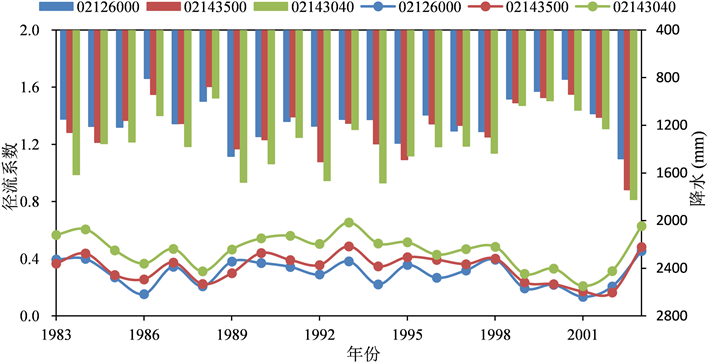Figure 3. Annual mean precipitation and runoff coefficient in the three study basinsTable 4. The observed precipitation and runoff during the dry years (1999-2001) and the wet year (2003) in the three study basinsTable 5. The variation of simulated runoff in the dry years (1999-2001) and wet year (2003) under the constant and time-varying model parameters schemes

1) 将参数考虑为气候因子的函数型式能明显提高径流的模拟精度，率定期NSE可提高18%，检验期可提高17%，且峰值模拟更优，有利于准确的径流预报。

2) 径流对降水的变化非常敏感，以往气候变化研究中将参数视为常数会低估径流的变化，三个流域径流结果平均低估30%，而时变参数方案与实际情况更贴近，径流变化量误差降至7%。

Identifying Functional Form of Time-Varying Hydrological Model Parameters under Changing Environment[J]. 水资源研究, 2018, 07(04): 351-359. https://doi.org/10.12677/JWRR.2018.74039

1. 1. 刘春蓁, 占车生, 夏军, 等. 关于气候变化与人类活动对径流影响研究的评述[J]. 水利学报, 2014, 45(4): 379-385, 393. LIU Chunzhen, ZHAN Chensheng, XIA Jun, et al. Review on the influences of climate change and human activities on runoff. Journal of Hydraulic Engineering, 2014, 45(4): 379-385, 393. (in Chinese)

2. 2. DENG, C., LIU, P., WANG, D., et al. Temporal variation and scaling of parameters for a monthly hydrologic model. Journal of Hydrology, 2018, 558: 290-300. https://doi.org/10.1016/j.jhydrol.2018.01.049

3. 3. MERZ, R., PARAJKA, J., ANDBLÖSCHL, G. Time stability of catchment model parameters: Implications for climate impact analyses. Water Resources Research, 2011, 47(2): 2144-2150. https://doi.org/10.1029/2010WR009505

4. 4. DENG, C., LIU, P., GUO, S., et al. Identification of hydrological model parameters variation using ensemble Kalman filter. Hydrology and Earth System Sciences, 2016, 22(5): 1-39.

5. 5. 张恭肃, 杨小柳, 安波. 确定性水文预报模型的实时校正[J]. 水文, 1987(1): 9-14. ZHANG Gongshu, YANG Xiaoliu, AN Bo. Real-time correction of deterministic hydrological forecasting model. Journal of China Hydrology, 1987(1): 9-14. (in Chinese)

6. 6. 熊立华, 郭生练, 付小平, 等. 两参数月水量平衡模型的研制和应用[J]. 水科学进展, 1996(S1): 80-86. XIONG Lihua, GUO Shenglian, FU Xiaoping, et al. Two-parameter monthly water balance model and its application. Advance in Water Science, 1996(S1): 80-86. (in Chinese)

7. 7. 张洪刚, 郭生练, 徐德龙, 等. 汉江流域半分布式两参数月水量平衡模型[J]. 人民长江, 2008, 39(17): 43-45. ZHANG Honggang, GUO Shenglian, XU Delong, et al. Semi-distributed two-parameter monthly water balance model in Hanjiangriver basin. Yangtze River, 2008, 39(17): 43-45. (in Chinese)

8. 8. 乐通潮, 张万昌. 双参数月水量平衡模型在汉江流域上游的应用[J]. 资源科学, 2004, 26(6): 97-103. LE Tongchao, ZHANG Wanchang. A two-parameter water balance model and its application in Hanjiangriver basin. Resources Science, 2004, 26(6): 97-103. (in Chinese)

9. 9. EVENSEN, G. Sequential data assimilation with a nonlinear quasi-geostrophic model using Monte Carlo methods to forecast error statistics. Journal of Geophysical Research-Oceans, 1994, 99(C5): 10143-10162. https://doi.org/10.1029/94JC00572

10. 10. FENG, M., LIU, P., GUO, S., et al. Deriving adaptive operating rules of hydropower reservoirs using time-varying parameters generated by the EnKF. Water Resources Research, 2017, 53(8): 6885-6907. https://doi.org/10.1002/2016WR020180

11. 11. 王卫光, 李进兴, 魏建德, 等. 基于蒸散发数据同化的径流过程模拟[J]. 水科学进展, 2018, 29(2): 159-168. WANG Weiguang, LI Jinxing, WEI Jiande, et al. Runoff simulation by hydrological model based on the assimilated evapotranspiration. Advances in Water Science, 2018, 29(2): 159-168. (in Chinese)

12. 12. DUAN, Q., SCHAAKE, J., ANDRéassian, V., et al. Model Parameter Estimation Experiment (MOPEX): An overview of science strategy and major results from the second and third workshops. Journal of Hydrology, 2005, 320(1): 3-17.